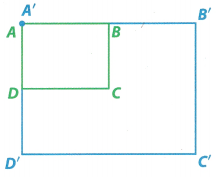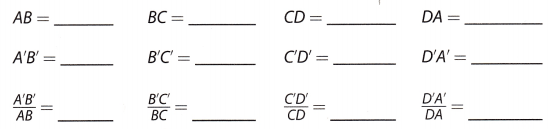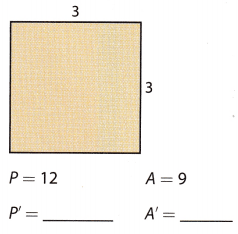# Texas Go Math Grade 8 Lesson 13.3 Answer Key Dilations and Measurement

Refer to our Texas Go Math Grade 8 Answer Key Pdf to score good marks in the exams. Test yourself by practicing the problems from Texas Go Math Grade 8 Lesson 13.3 Answer Key Dilations and Measurement.

## Texas Go Math Grade 8 Lesson 13.3 Answer Key Dilations and Measurement

Exploring Dilations and Measurement

The blue rectangle is a dilation (enlargement) of the green rectangle.A. Using a centimeter ruler, measure and record the length of each side of both rectangles. Then calculate the ratios of all pairs of corresponding sides.Dilation means transformation which is used to make the object into smaller size or larger size.
The length of AB = 4cm
The length of BC = 3cm
The length of CD = 4cm
The length of DA = 3cm
The length of AB’ = 8cm
The length of B’C’ = 6cm
The length of C’D’ = 8cm
The length of D’A’ = 8cm
A’B’/AB = 8/4 = 4cm
B’C’/BC = 6/3 = 3cm
C’D’/CD = 8/4 = 4cm
D’A’/DA = 6/3 = 3cm
What is true about the ratios that you calculated?
Answer: The blue rectangle is double the green rectangle. The ratio of the blue rectangle and the green rectangle is calculated then we get the length of the green rectangle.

What scale factor was used to dilate the green rectangle to the blue rectangle?
The scale factor is used to dilate the green rectangle to the blue rectangle.
Scalar factor = A’B’/AB = 8/4 = 4cm
Scalar factor = B’C’/BC = 6/3 = 3cm
Scalar factor = C’D’/CD = 8/4 = 4cm
Scalar factor = D’A’/DA = 6/3 = 3cm

How are the side lengths of the blue rectangle related to the side lengths of the green rectangle?
The sides of the blue rectangle are double times the green rectangle.
The lengths of the green rectangle.
The length of AB = 4cm
The length of BC = 3cm
The length of CD = 4cm
The length of DA = 3cm
The lengths of the blue rectangle.
The length of AB’ = 8cm
The length of B’C’ = 6cm
The length of C’D’ = 8cm
The length of D’A’ = 8cm

B. What is the perimeter of the green rectangle? _______________
What is the perimeter of the blue rectangle? _______________
How is the perimeter of the blue rectangle related to the perimeter of the green rectangle?
i. The formula for the perimeter of the rectangle = 2(l + w)
The length of the green rectangle = 4cm
The width of the green rectangle = 3cm.
P = 2(4 + 3)
P = 14
The perimeter of the green rectangle = 14cm
ii. The formula for the perimeter of the rectangle = 2(l + w)
The length of the blue rectangle = 8cm
The width of the blue rectangle = 6cm.
P = 2(8 + 6)
P = 28
The perimeter of the blue rectangle = 28cm
iii. The perimeter of the green rectangle = 14cm
The perimeter of the blue rectangle = 28cm
The perimeter of the green rectangle is half of the blue rectangle.

C. What is the area of the green rectangle? _______________
What is the area of the blue rectangle? _______________
How is the area of the blue rectangle related to the area of the green rectangle?
i. The formula for the area of the rectangle = l x w
The length of the green rectangle = 4cm
The width of the green rectangle = 3cm.
A = 4 x 3
A = 12
The area of the green rectangle = 12 square units.
ii. The formula for the perimeter of the rectangle = l x w
The length of the blue rectangle = 8cm
The width of the blue rectangle = 6cm.
P = 8 x 6
P = 48
iii. The area of the green rectangle = 12 square units
The area of the blue rectangle = 48 square units.
The area of the green rectangle is 4 times the blue rectangle.

Reflect

Question 1.
Make a Conjecture The perimeter and area of two shapes before and after dilation are given. How are the perimeter and area of a dilated figure related to the perimeter and area of the original figure?For the first shape
The perimeter of the original figure = 8
The perimeter of the dilation figure = 16
The perimeter of the original figure is half the dilation figure.
The area of the original figure = 4 square units.
The area of the dilation figure = 16 square units.
The area of the dilation is 4 times more than the original figure.
For the original figure
The perimeter of the original figure = 30
The perimeter of the dilation figure = 5
The perimeter of the original figure is 6 times the dilation figure.
The area of the original figure = 54 square units.
The area of the dilation figure = 1.5 square units.
The area of the dilation is 36 times more than the original figure.

Question 2.
Johnson Middle School is selling mouse pads that are replicas of a student’s award-winning artwork. The rectangular mouse pads are dilated from the original artwork and have a length of 9 inches and a width of 8 inches. The perimeter of the original artwork is 136 inches. What is the area of the original artwork?
Given that,
The perimeter of the original artwork = 136inches.
The formula for the perimeter of the rectangle = 2(l +b)
The length of the dilated mouse = 9inches
The Width of the dilated mouse = 8 inches.
The length of the original mouse = 9 x 4 = 36inches.
The width of the original mouse = 8 x 4 = 32 inches.
= 2(36 + 32) = 136 inches.
Area of the rectangle = l x b
= 36 x 32 = 1152inches.
The area of the original artwork = 1152 inches.

Find the perimeter and area of the image after dilating the figures shown with the given scale factor. (Explore Activity and Example 1)

Question 1.
Scale factor = 5Given that,
The perimeter of the square = 12
Scale factor = 3.
The perimeter of the P’ = P x square factor
= 12 x 3
The perimeter of the P’ = 36
The area of the P = 9
The area of the P’ = 9 xsquare of scale factor = 12 x 3² = 108

Question 2.
Scale factor = $$\frac{3}{4}$$Given that,
The perimeter of the square = 48
Scale factor = ¾ = 0.75
Perimeter of the P’ = P x square factor
= 48 x 0.75 = 36
Perimeter of the P’ = 36
The area of the P = 128
The area of the P’ = 128 x square of scale factor = 128 x 0.75² = 71.68

A group of friends is roping off a soccer field in a back yard. A full-size soccer field is a rectangle with a length of 100 yards and a width of 60 yards. To fit the field in the back yard, the group needs to reduce the size of the field so its perimeter is 128 yards. (Example 1)

Question 3.
What is the perimeter of the full-size soccer field?
The length of the soccer field = 100 yards.
The width of the soccer field = 60 yards.
The perimeter of the rectangle = 2(l + b)
= 2(100 + 60)
= 320yards
The perimeter of the full-size soccer field = 320 yards.

Question 4.
What is the scale factor of the dilation?
The dilation soccer field perimeter = 128 yards.
The formula for the perimeter of the rectangle = 2(l + b)
The length of the soccer field = 100 yards.
The width of the soccer field = 60 yards
The length of the dilated field = 42 yards.
The length of the dilated field is 2.4 times less than the original length.
The width of the dilated field = 22 yards
The width of the dilated field is 2.7 times less than the original width.
Scale factor of the dilation = larger length/smaller length = 100/42 = 2.38
The scale factor of the dilation = 21/50

Question 5.
What is the area of the soccer field in the back yard?.
The formula for the area of the rectangular soccer field in the backyard = l x b
The length of the soccer field in the backyard = 100 yards.
The width of the soccer field in the backyard = 60 yards.
= 100 x 60
= 6000 square yards.
The area of the rectangular soccer field in the backyard = 6000 square yards.

Essential Question Check-In

Question 6.
When a rectangle is dilated, how do the perimeter and area of the rectangle change?
The perimeter of the dilated rectangular soccer field in the backyard = 128 yards.
The perimeter of the original rectangular soccer field in the backyard = 2(100 + 60) = 130 yards
The area of the rectangular soccer field in the backyard = 6000 square yards.
The area of the dilated rectangle = 42 x 22 = 924.
The perimeter and area of the rectangle are less than the original rectangle.

Question 7.
When you make a photocopy of an image, is the photocopy a dilation? What is the scale factor? How do the perimeter and area change?
Assume that the image is in the shape of a rectangle.
Dilation length = 4cm
Perimeter of the dilation photocopy = 2(4 + 2) = 12
The area of the dilation photocopy = l x b = 4 x 2 = 8 square cm
Scale factor 4/2 = 2.

Question 8.
Problem Solving The universally accepted film size for movies has a width of 35 millimeters. If you want to project a movie onto a square sheet that has an area of 100 square meters, what is the scale factor that is needed for the projection of the movie? Explain.
The film size for movies has a width = 35 millimeters.
The movie onto a square sheet that has an area = 100 square meters.
Width od the square sheet = 4a = 100/4 = 25mm
Scale factor = 35/25 = 7/5.

Question 9.
The perimeter of a square is 48 centimeters. If the square is dilated by a scale factor of 0.75, what is the length of each side of the new square?
Given that,
The perimeter of a square = 48 centimeters
48/4 = 12.
The formula for the perimeter of the square = 4a
The square is dilated by a scale factor = 0.75,
The length of each side of a square = 12.
The length of the new square = 12 x 0.75 = 9 cm.

Question 10.
The screen of an eReader has a length of 8 inches and a width of 6 inches. Can the page content from an atlas that measures 19 inches by 12 inches be replicated in the eReader? If not, propose a solution to move the atlas content into the eReader format.
The screen of an eReader length = 8 inches.
The screen of an eReader width of 6 inches.
The page content from an atlas that length = 19 inches.
The page content from an atlas that breadth = 12 inches
The page content of the atlas cannot be replicated on the eReader.

Question 11.
Represent Real-World Problems There are 64 squares on a chessboard. Each square on a tournament chessboard measures 2.25 × 2.25 inches. A travel chessboard is a dilated replica of the tournament chessboard using a scale factor of $$\frac{1}{3}$$.

a. What is the size of each square on the travel chessboard?
Answer: The size of each square on the travel chessboard is 2.25 x 2.25 = 5.062.

b. How long is each side of the travel board?
Answer: Each side of the travel board = 2.25 inches.

c. How much table space do you need to play on the travel chessboard?
The number of squares on the chessboard = 64.
The size of each square = 5.062.
The tablespace on the travel chessboard = 64 x 5.062 = 323.968.

Question 12.
Draw Conclusions The legs of a right triangle are 3 units and 4 units long. Another right triangle is dilated from this triangle using a scale factor of 3. What are the side lengths and the perimeter of the dilated triangle?
The legs of a right rectangle = 3 units and 4 units.
Scale factor = 3.
The side lengths of the dilated triangle = 3 x 3 = 9
And 4 x 3 = 12
The side lengths of the dilated triangle = 9 units and 12 units
Perimeter of the dilated triangle = P = a + b + √(a²+ b²)
A = 9units
B = 12 units
P = 9 + 12 + √(9²+ 12²)
= 9 + 12 + 153
Perimeter of the dilated triangle = 174 units.

H.O.T. Focus on Higher Order Thinking

Question 13.
Critique Reasoning Rectangle W’X’Y’Z’ below is a dilation of rectangle WXYZ. A student calculated the area of rectangle W’X’Y’Z’ to be 36 square units. Do you agree with this student’s calculation? If not, explain and correct the mistake.Given that
The area of the W’X’Y’Z’ is 36 square units.
The area of the WXYZ is 12 x 9 = 108.
The length of the original rectangle = 12
The width of the original rectangle = 9
The length of the dilation rectangle = 4
It means 12/4 = 3
The dilation rectangle is 3 times less than the original rectangle.
The width of the dilation rectangle = 9/3 = 3
The area of the W’X’Y’Z’ is l x b = 4 x 3 = 12
The area of the W’X’Y’Z’ to be 36 square units is wrong.

Question 14.
Multistep Rectangle A’B’C’D’ is a dilation of rectangle ABCD, and the scale factor is 2. The perimeter of ABCD is 18 mm. The area of ABCD is 20 mm2.
a. Write an equation for, and calculate, the perimeter of A’B’C’D’.
The perimeter of ABCD is 18 mm.
Rectangle A’B’C’D’ is a scale factor of 2.
The perimeter of A’B’C’D’ = 18 x 2 = 32mm

b. Write an equation for, and calculate, the area of A’B’C’D’.
The area of ABCD is 20 mm².
The area of the A’B’C’D’ = ABCD area x square of scale factor.
= 20 x 2²
= 80mm²

c. The side lengths of both rectangles are whole numbers of millimeters. What are the side lengths of ABCD and A’B’C’D’?